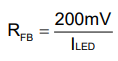# MP3362 Reference design in data sheet

3362 ref design: It shows a 10K resistor (R4) in series with the RFB (R5) resistor. Given the data sheet formula for calculating current (RFB) what is the purpose of R4 in series with R5.

Hello Sparker,

The 10k is used as an Rt for part stabilty and noise immunity. To calculated ILed, please use the following equation:Regards,
Vinh Tran